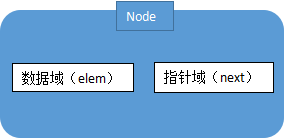# 什么是线性表？数据结构之线性表讲解!

## 顺序表

 null arr0=W arr1=3 arr2=C arr3=S arr4=C arr5=H arr6=o arr7=o arr8=l null null null null null null null null null null

## 链式表

 arr1 =W 下一个目标是 第三格 null arr2 =3 下一个目标是 第五格 null arr3 =C  下一个目标是 第六格 arr4=/0 这里是链表尾部 null## 链表的C语言实现：单链表

``````#include <stdio.h>
#include <stdlib.h>
int  elem;
//查找结点的函数，elem为目标结点的数据域的值
//更新结点的函数，newElem为新的数据域的值
int main() {
//初始化链表（1，2，3，4）
printf("初始化链表为：\n");
display(p);

printf("在第4的位置插入元素5：\n");
p=insertElem(p, 5, 4);
display(p);

printf("删除元素3:\n");
p=delElem(p, 3);
display(p);

printf("查找元素2的位置为：\n");
printf("没有该元素");
}else{
}
printf("更改第3的位置的数据为7:\n");
p=amendElem(p, 3, 7);
display(p);

return 0;
}
//生成链表
for (int i=1; i<5; i++) {
a->elem=i;
a->next=NULL;
temp->next=a;
temp=temp->next;
}
return p;
}
//首先找到要插入位置的上一个结点
for (int i=1; i<add; i++) {
if (temp==NULL) {
printf("插入位置无效\n");
return p;
}
temp=temp->next;
}
//创建插入结点c
c->elem=elem;
//向链表中插入结点
c->next=temp->next;
temp->next=c;
return  p;
}
//遍历到被删除结点的上一个结点
for (int i=1; i<add; i++) {
temp=temp->next;
}
temp->next=temp->next->next;//删除某个结点的方法就是更改前一个结点的指针域
free(del);//手动释放该结点，防止内存泄漏
return p;
}
int i=1;
while (t->next) {
t=t->next;
if (t->elem==elem) {
return i;
}
i++;
}
return -1;
}
temp=temp->next;//tamp指向首元结点
//temp指向被删除结点
for (int i=1; i<add; i++) {
temp=temp->next;
}
temp->elem=newElem;
return p;
}
//只要temp指针指向的结点的next不是Null，就执行输出语句。
while (temp->next) {
temp=temp->next;
printf("%d",temp->elem);
}
printf("\n");
}``````

## 小结：顺序表和链式表的优缺点比较

 顺序表 链式表 内存使用 连续的一块空间，数据相邻，通过物理相邻的方式实现数据之间的联系 。 因此不能动态调整长度 （内存使用率较低） 每个数据节点不一定相邻，通过指针的方式实现数据之间的联系 因此可以调整动态长度 删除 可以删除最后一个数据，删除其他位置的数据的时候需要将相应的数据前移 （删除成本高） 数据可以随意删除，只需要将前一个节点的指针指向后一个节点 插入 可以在顺序表最后添加一个新的数据，在其他位置插入数据的时候需要将相应的数据后移再插入 （插入成本高） 数据可以随意插入，只需要将前一个节点的指针指向新的节点，再将新的节点的指针指向下一个节点 元素查询 索引与内存偏移正相关，只需要知道索引就可以得到相应的内存地址，只需要执行一次数据访问就可以得到数据 需要一个节点一个节点的查找才能得到数据，当节点在末尾的时候需要遍历整个链表才能得到数据。 （查询成本高）

1 人点赞